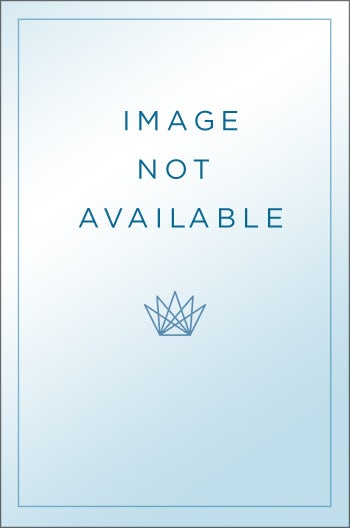Deductive Systems and the Decidability Problem for Hybrid Logics

Jagiellonian University PressPub Date: October 2014

ISBN: 9788323336952

232 Pages

Format: Paperback

List Price: \$55.00£46.00

# Deductive Systems and the Decidability Problem for Hybrid Logics

Jagiellonian University Press

This book stands at the intersection of two topics: the decidability and computational complexity of hybrid logics, and the deductive systems designed for them. Hybrid logics are here divided into two groups: standard hybrid logics involving nominals as expressions of a separate sort, and non-standard hybrid logics, which do not involve nominals but whose expressive power matches the expressive power of binder-free standard hybrid logics.The original results of this book are split into two parts. This division reflects the division of the book itself. The first type of results concern model-theoretic and complexity properties of hybrid logics. Since hybrid logics which we call standard are quite well investigated, the efforts focused on hybrid logics referred to as non-standard in this book. Non-standard hybrid logics are understood as modal logics with global counting operators (M(En)) whose expressive power matches the expressive power of binder-free standard hybrid logics. The relevant results comprise: 1. Establishing a sound and complete axiomatization for the modal logic K with global counting operators (MK(En)), which can be easily extended onto other frame classes, 2. Establishing tight complexity bounds, namely NExpTime-completeness for the modal logic with global counting operators defined over the classes of arbitrary, reflexive, symmetric, serial and transitive frames (MK(En)), MT(En)), MD(En)), MB(En)), MK4(En)) with numerical subscripts coded in binary. Establishing the exponential-size model property for this logic defined over the classes of Euclidean and equivalential frames (MK5(En)), MS5(En)).Results of the second type consist of designing concrete deductive (tableau and sequent) systems for standard and non-standard hybrid logics. More precisely, they include: 1. Devising a prefixed and an internalized tableau calculi which are sound, complete and terminating for a rich class of binder-free standard hybrid logics. An interesting feature of indicated calculi is the nonbranching character of the rule (¬D), 2. Devising a prefixed and an internalized tableau calculi which are sound, complete and terminating for non-standard hybrid logics. The internalization technique applied to a tableau calculus for the modal logic with global counting operators is novel in the literature, 3. Devising the first hybrid algorithm involving an inequality solver for modal logics with global counting operators. Transferring the arithmetical part of reasoning to an inequality solver turned out to be sufficient in ensuring termination.The book is directed to philosophers and logicians working with modal and hybrid logics, as well as to computer scientists interested in deductive systems and decision procedures for logics. Extensive fragments of the first part of the book can also serve as an introduction to hybrid logics for wider audience interested in logic.The content of the book is situated in the areas of formal logic and theoretical computer science with some elements of the theory of computational complexity.
I consider the book Deductive Systems and the Decidability Problem for Hybrid Logic as () a very reliable survey on the issue of computational complexity of hybrid logics, which, additionaly, makes a significant contribution to this area of research."Worth stressing is the fact that the author shows great expertise in the topic referring to extensive literature."(The book) presents the syntax, the semantics, the expressive power and the computational complexity of the satisfiability problem for hybrid logics. The author proves the results on the axiomatizability and the computational complexity of selected non-standard hybrid logics. Apart from the indicated important metalogical problems, the book contains a whole range of theorems on the existence of the finite model property for selected hybrid logics."The results of the second part of the book concern deductive systems (tableau and sequent) for standard and non-standard hybrid logics. The results reported in this part comprise devising two tableau calculi – internalized () and prefixed () – and investigating their soundness and completeness for a wide class of both standard and non-standard hybrid logics."The book is devoted to several very interesting issues. The author provides a general introduction to hybrid logics. Since he is interested in the problem of decidability for these logics, he selects some of them, presents all necessary tools used in the proofs and, finally, he establishes the complexity class for a given logic. On this occasion, considering various tableau and sequent calculi for these logics, he investigates many proof-theoretical issues, in particular several blocking mechanisms and restrictions that can be put on the application of rules. Marek Nasieniewski, Nicolaus Copernicus University